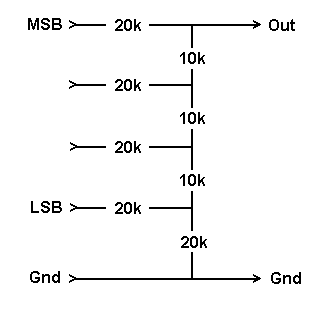Daqarta
Data AcQuisition And Real-Time Analysis
Scope - Spectrum - Spectrogram - Signal Generator
Software for WindowsThe following is from the Daqarta Help system:

# DAC (Digital-to-Analog Converter)

This device (also known as a D/A) converts binary numbers into equivalent scaled voltages. (A complimentary ADC or A/D converts analog signals into digital values, and a codec (coder-decoder) has both in a single package.)

The number of possible digital input values (and hence analog output voltages) is 2^N, where N is the number of bits of the DAC. A 16-bit DAC thus has 65536 possible output values; and 8-bit DAC only 256.

You could make a simple DAC with a bunch of weighted resistors connected to the digital outputs of any parallel port such as a printer port. The smallest resistor would connect to the most significant bit, and each adjacent bit would get twice the value of the one before it. Here is a crude 4-bit DAC:This approach is rarely used inside real DAC chips, due to problems with the large resistor values needed as the number of bits increases, and the general difficulty in getting exact multiples. Instead, there are "ladder" schemes that use the same values over and over. The exact values don't matter as much as their relative matching, and this turns out to be much easier to integrate on a chip. A simple ladder version of the above would be:The five 20K resistors are all matched, and are exactly twice the value of the three 10K resistors. This circuit is known as an "R-2R ladder DAC". It can be extended to 8 bits by following the same pattern. Note that there is always one more 2R value and one less R value than the number of bits, so an 8-bit DAC would have 9 x 20K resistors and 7 x 10K. The DaquinOscope macro mini-app for Arduino boards uses just such an 8-bit DAC, and includes board layouts and construction details.

If you actually build this circuit or the prior one, note that they assume that all of the logic 1 driving voltages match (say, 5.00 volts), and that logic 0 is really 0 volts. That's not guaranteed for older TTL-type digital port outputs, but for the simple 4-bit systems shown here it would be good enough. Modern CMOS outputs (such as those on the Arduino) are much better, such that they can easily drive 8-bit ladders.

Current sound cards typically use another approach to DAC construction, which is like the reverse of a delta-sigma ADC. The digital output data stream is digitally converted from normal sound card sample rates and encoding, up to very high rates and with a special encoding. It is then sent to a single-bit DAC, which is really just an on-off switch, and finally to a simple low-pass output filter.

Since there are no resistors to match, this approach can be made very accurate for low distortion. Also, the analog output filter can be very simple yet achieve superb performance at reconstructing the signal, since it only needs to remove very high frequency output steps, far removed from the audio range.GO: